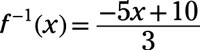##### Pre-Calculus: 1001 Practice Problems For Dummies (+ Free Online Practice)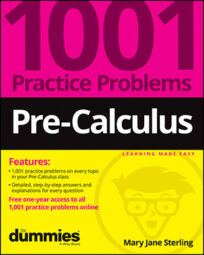A function is a special type of rule or relationship. The difference between a function and a relation is that a function has exactly one output value (from the range) for every input value (from the domain).

Functions are very useful when you’re describing trends in business, heights of objects shot from a cannon, times required to complete a task, and so on. Functions have some special properties and operations that allow for investigation into what happens when you change the rule.

In pre-calculus, you’ll work with functions and function operations in the following ways:

• Writing and using function notation

• Determining the domain and range of different types of functions

• Recognizing even and odd functions

• Checking on whether a function is one-to-one

• Finding inverses of one-to-one functions

• Performing the basic operations on functions and function rules

• Working with the composition of functions and the difference quotient

Don’t let common mistakes trip you up; keep in mind that when working with functions, your challenges will include

• Following the order of operations when evaluating functions

• Determining which values need to be excluded from a function’s domain

• Working with negative signs correctly when checking for even and odd functions

• Being sure a function is one-to-one before trying to determine an inverse

• Correctly applying function rules when performing function composition

• Raising binomials to higher powers and including all the terms

## Practice problems

1. Find the domain and range for the function.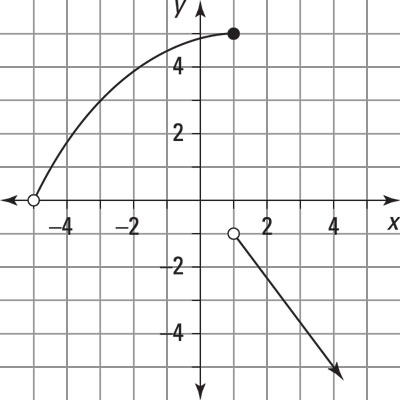Credit: Illustration by Thomson Digital

Answer: domain: –5 < x; range: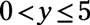or y < –1.

The domain is the set of x values, and the range is the set of y values for which the function is defined. In this case, x is defined for all real numbers greater than −5. You don’t include −5 because there’s an open dot on −5 in the graph. If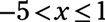then the range isIf x > 1, then the range is all real numbers less than −1. So the range isor y < –1.

2. Find the inverse of the function: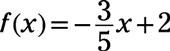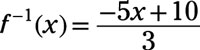Change f(x) to y: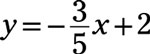Interchange x and y: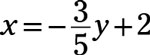Now solve for y: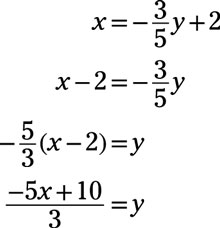Rename y as f–1(x):